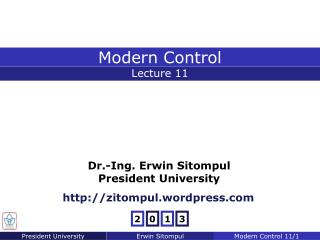Download PresentationHomework 9

# Homework 9

Download Presentation## Homework 9

- - - - - - - - - - - - - - - - - - - - - - - - - - - E N D - - - - - - - - - - - - - - - - - - - - - - - - - - -
##### Presentation Transcript

1. Chapter 10 Optimal Control Homework 9 Consider again the control system as given before, described by Assuming the linear control law Determine the constants k1 and k2 so that the following performance index is minimized Consider only the case where the initial condition is x(0)=[c 0]T and the undamped natural frequency (ωn) is chosen to be 2 rad/s. • Recall again the standard form of a second order transfer function (FCS) • Calculate the transfer function of the system if compensated with k • Determine the value of corresponding k (k1 or k2?) to obtain ωn as requested

2. Chapter 10 Optimal Control Solution of Homework 9 Substituting the state feedback and finding the transfer function,

3. Chapter 10 Optimal Control Solution of Homework 9

4. Chapter 10 Optimal Control Algebraic Riccati Equation • Consider again the n-dimensional state space equations: with the following performance index to be minimized: : symmetric, positive semidefinite • The control objective is to construct a stabilizing linear state feedback controller of the form u(t) = –Kx(t) that at the same time minimizes the performance index J. The state feedback equation u(t) = –Kx(t) is also called the “control law.”

5. Chapter 10 Optimal Control Algebraic Riccati Equation • First, assume that there exists a linear state feedback optimal controller, such that the optimal closed-loop system: is asymptotically stable. • Then, there exists a Lyapunov Function V = xT(t)Px(t) with a positive definite matrix P, such that dV/dt evaluated on the trajectories of the closed-loop system is negative definite. • The synthesis of optimal control law involves the finding of an appropriate Lyapunov Function, or equivalently, the matrix P.

6. Chapter 10 Optimal Control Algebraic Riccati Equation • The appropriate matrix P is found by minimizing: • For unconstrained minimization, Optimal Solution If u(t) = –Kx(t) is so chosen that min{f(u(t)) = dV/dt + xT(t)Qx(t) + uT(t)Ru(t)} = 0 for some V = xT(t)Px(t), Then the controller using u(t) as control law is an optimal controller.

7. Chapter 10 Optimal Control Algebraic Riccati Equation • The differentiation yields: if P symmetric

8. Chapter 10 Optimal Control Algebraic Riccati Equation • Hence, incorporating the fact that P and R are symmetric, the optimal control law can be written as: or

9. Chapter 10 Optimal Control Algebraic Riccati Equation • We now need to perform the “Second Derivative Test” to find out whether u*(t) is a solution that minimizes f(u(t)). Second Derivative Test • If f’(x) = 0 and f”(x) > 0 then f has a local minimum at x • If f’(x) = 0 and f”(x) < 0 then f has a local maximum at x • If f’(x) = 0 and f”(x) = 0 then no conclusion can be drawn • Performing the “Second Derivative Test”, • If the weight matrix R is chosen to be a positive definite matrix, then the optimal solution u*(t) is indeed a solution that minimizes f(u(t)).

10. Chapter 10 Optimal Control Algebraic Riccati Equation • Now, the appropriate matrix P must be found, in order to obtain the optimal closed-loop system in the form of: • The optimal controller with matrix P minimizes the cost function f(u(t)), and will yield:  • After some substitutions of x(t) and later u*(t),

11. Chapter 10 Optimal Control Algebraic Riccati Equation • After regrouping, we will obtain: • The equation above should hold for any x(t), which implies that: Algebraic Riccati Equation (ARE) • After solving the ARE for P, the optimal control law given by: can be applied to the linear system of

12. Chapter 10 Optimal Control Example 1: Algebraic Riccati Equation Consider the following model: along with the performance index: Find the optimal control law for the system. The matrices are: The ARE is solved as:

13. Chapter 10 Optimal Control Example 1: Algebraic Riccati Equation The control law is: The optimal closed-loop system is described by:

14. Chapter 10 Optimal Control Example 2: Algebraic Riccati Equation Consider the following continuous-time system: Design an optimal controller that minimizes with give weight to x1(t), no restriction for x2(t)

15. Chapter 10 Optimal Control Example 2: Algebraic Riccati Equation Pis found by solving the ARE:

16. Chapter 10 Optimal Control Example 2: Algebraic Riccati Equation Three equations can be obtained: Thus, the optimal gain is given by: The requested control law is:

17. Chapter 10 Optimal Control Homework 10 The regulator shown in the figure below contains a plant that is described by and has a performance index Determine The Riccati matrix P The state feedback matrix k The closed-loop eigenvalues

18. Chapter 10 Optimal Control Homework 10A Consider the system described by the equations Determine the optimal control u*(t) which minimizes the following performance index. (Hint: User Algebraic Riccati Equation.)